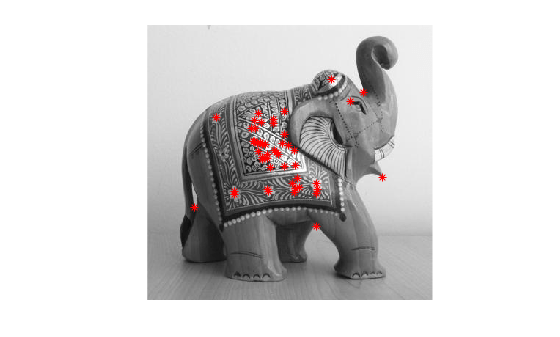# keyPointsToStruct

Convert OpenCV `KeyPoints` object to MATLAB structure

## Syntax

``mlstruct = keyPointsToStruct(keypoints)``

## Description

example

````mlstruct = keyPointsToStruct(keypoints)` converts an OpenCV `KeyPoints` object to a MATLAB® structure.```

## Examples

collapse all

This example shows how to use the prebuilt MATLAB interface for the OpenCV function `cv::Fast` in MATLAB to detect keypoints in an image. Additionally, use the `keyPointsToStruct` utility function to write the keypoints returned by the OpenCV `cv::Fast` function to a MATLAB structure.

Add the MATLAB interface to OpenCV package names to the import list.

```import clib.opencv.*; import vision.opencv.util.*;```

Read an image into the MATLAB workspace.

`img = imread("elephant.jpg");`

Create MATLAB interface objects for the OpenCV `MatND` and `InputArray` classes to store the input image.

`[inputMat,inputArray] = createMat(img);`

Create a MATLAB interface object for the OpenCV `KeyPoint` vector by using the `clibArray` function.

`keyPointsVec = clibArray("clib.opencv.cv.KeyPoint",0);`

Specify the parameters for computing keypoints using the FAST detector.

```threshold = 100; nonmaxSuppression = true;```

Compute keypoints in the image by calling the OpenCV function `cv::FAST` in MATLAB.

`cv.FAST(inputArray,keyPointsVec,threshold,nonmaxSuppression);`

Convert the `KeyPoints` object returned by the OpenCV function into a MATLAB structure.

`mlstruct = keyPointsToStruct(keyPointsVec);`

Inspect the fields in the output MATLAB structure.

`mlstruct`
```mlstruct = struct with fields: Location: [48x2 double] Scale: [48x1 double] Metric: [48x1 double] Misc: [48x1 double] Orientation: [48x1 double] ```

Display the input image and plot the detected keypoints.

```figure imshow(img) hold on plot(mlstruct.Location(:,1),mlstruct.Location(:,2),"*r") hold off```## Input Arguments

collapse all

OpenCV `KeyPoints` class, specified as a MATLAB interface object. This interface object is a representation of the `KeyPoints` class returned by any of the OpenCV functions for keypoint detection.

## Output Arguments

collapse all

Keypoints detected using the OpenCV function, returned as a MATLAB structure with fields `Location`, `Scale`, `Metric`, `Misc`, and `Orientation`.

 Fields Description `Location` x and y-coordinates of the keypoints. `Scale` Diameter of the neighborhood region around the keypoints. `Metric` Strength of the keypoints. `Orientation` Orientation of the keypoints.

## Version History

Introduced in R2021b